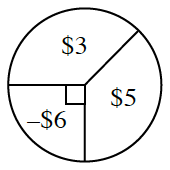### Home > INT2 > Chapter 3 > Lesson 3.2.2 > Problem3-84

3-84.

The probability of winning $3$ on the spinner at right is equal to the chance of winning $5$. Calculate the expected value for one spin. Is this game fair?

Refer to the Math Notes box in Lesson 3.2.1.

Part of the calculation for the expected value is $\3\left(\frac{135}{360}\right)$.

Is the game fair?
That means the amount you win is equal to the amount you lose, or the expected value is $0$.